# Calculated Density (NX-19)

## Options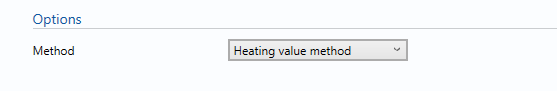### Method

• Specific gravity method
• Methane-Gravity method
• Heating value method

Select the type of method to be used in calculation of the density (NX-19).

## Coverage Factor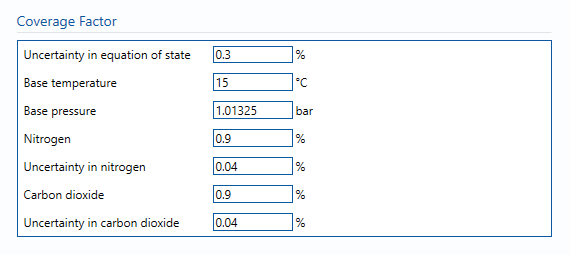Uncertainty in equation of state – Uncertainty in the equation of state. Guidelines on determining this value can be found in the relevant standard.

Note: that the uncertainties given in AGA8:1994, Figure 1 are only valid for the “normal” range of compositions given in the standard; for compositions in the “extended” range the uncertainty will be higher, but the standard does not specify how much higher. Engineering judgement should be used here, however it is recognised that this is difficult at best, due to lack of data.

Base temperature – the temperature at base or standard conditions.

Base pressure – the pressure at base or standard conditions.

Nitrogen – the percentage nitrogen in the gas stream.

Uncertainty in nitrogen – the uncertainty in the nitrogen measurement.

Carbon dioxide – the percentage of carbon dioxide in the gas stream.

Uncertainty in carbon dioxide – the uncertainty in the carbon dioxide measurement.

## Uncertainty Budget

The uncertainty calculation is detailed in the uncertainty budget table giving a break down of how the overall uncertainty is calculated. The values input into the uncertainty budget are taken from the module inputs and calculations from other blocks along with the uncertainty in the equation of state as input in this block.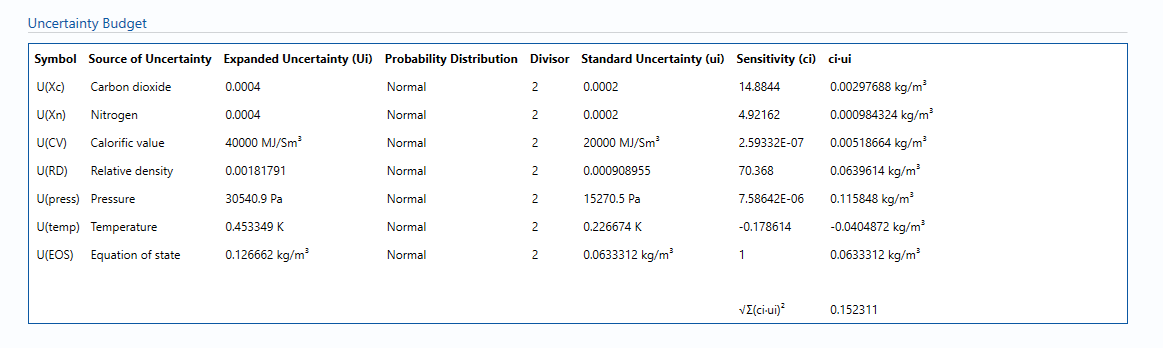Uncertainties associated with these values are taken in as the Expanded Uncertainties. The Standard Uncertainty is calculated from dividing the Expanded Uncertainty by the coverage factor which is determined by the probability distribution that best suits that uncertainty component.

The standard uncertainty is then multiplied by the sensitivity value then squared. This is done for each parameter that contributes to the overall uncertainty in the Line Density. The Standard Uncertainty in Line Density is the square root of the sum of each component variance as shown in the following equation: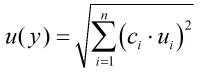### Calculated Uncertainty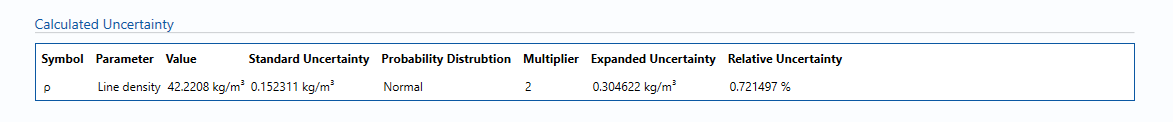The Expanded Uncertainty is the Standard Uncertainty multiplied by the coverage factor (k). The coverage factor is defaulted to k = 2 (equivalent to a confidence level of approximately 95%).

The Relative Uncertainty is the Expanded Uncertainty divided by the Line Density .

Back to Uncertainty Modules